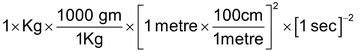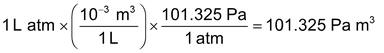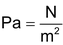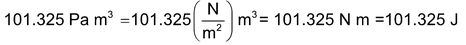# Introduction

## Some Basic Concept Of Chemistry of Class 11

Chemistry is the science of substances, their properties, structures and their transformation. As all objects in the universe are made of matter. Chemistry is the branch of the science which deals with the study of material object. Study of chemistry is very interesting which covers various aspects of our culture and environment.

All development in any science are based on scientific approach as in chemistry too. In order to achieve correct results, one has to rely upon the various skills connected with the measurements of quantities during a physical or chemical change. The degree of accuracy is closely linked with precision of the measuring instrument as well as on the skill of the person engaged in measurement. So we should be first familiar with some terminology used in chemistry.

## Physical Property:

The property which can be measured without changing the chemical composition of the substance known as physical property like mass, volume, density, refractive index etc.

### Chemical Property:

The property which can be evaluated at the cost of matter itself known as chemical property. For example combustible nature of hydrogen gas can be verified by burning of hydrogen. The sweet taste of sugar by consuming it.

### Units for Measurement

All physical quantities have to be measured. The value of a physical quantity is expressed as the product of the numerical value and the unit in which it is expressed.

### Fundamental Units:

Fundamental units are those units which can neither be derived from one another nor they can be further resolved into any other units.

The seven fundamental units of measurement in S.I. system.

 Name of unit Abbreviation Mass Kilogram Kg Length Meter m Temperature Kelvin K Amount of substance Mole Mol Time Second S Electric current Ampere A Luminous intensity Candela Cd

### Derived unit:

Some quantities are expressed as a function of more than one fundamental units known as derived units. For example velocity, acceleration, works, energy etc.

 Quantity with Symbol Unit (S.I.) Symbol Velocity (v Metre per sec ms−1 Area (A) Square metre m2 Volume (V) Cubic metre m3 Density (ρ) Kilogram m−3 Kg m−3 Energy (E) Joule (J) Kg m2s−2 Force (F) Newton (N) Kg ms−2 Frequency (ν) Hertz Cycle per sec Pressure (P) Pascal (Pa) Nm−2 Electrical charge Coulomb (C) A-s (ampere – second)

Units and Dimensional Analysis: Conversion of Units

The simplest way to carry out calculations that involve different units is to use dimensional analysis. In this method a quantity expressed in one unit is converted into an equivalent quantity with a different unit by using conversion factor which express the relationship between units:

Original quality×(conversion factor = equivalent quantity

(in former unit) (in other unit)

This is based on the fact that ratio of each fundamental quantity in one unit with their equivalent quantity in other unit is equal to one

For example in case of mass

1kilogram/2.205 pound = 1 = 1kilogram/1000 gm

So 1 kg = 2.205 pond = 1000 gm

In this way any derived unit first expressed in dimension and each fundamental quantities like mass length time are converted in other system of desired unit to work out the conversion factor.

For example: How unit of work / energy …………… joule in S.I. system related with unit erg in C.G.S system

Dimension of work = force × displacement = MLT-2 × L = ML2T-2

1 joule = 1 kg (1 metre)2 × (1see)-2⇒ 100 gm × (100)2× 1 em2× (1 sec)-2

⇒ 1000×10000 × 1 gm×1 cm2 × 1 sec-1

⇒ 1 joule = 107 erg

Similarity we can deduce other conversion factor for other quantity in different unit by the dimensional analysis method

Another interesting example is the conversion of litre – atmosphere to joule (the SI unit of energy) by multiplying with two successive unit factors. Thus,Knowing that, we can writeHence 1 L atm = 101.325 J

Matter

Anything that exhibits inertia known as matter. The quantity of matter is its mass. e.g. chalk table.